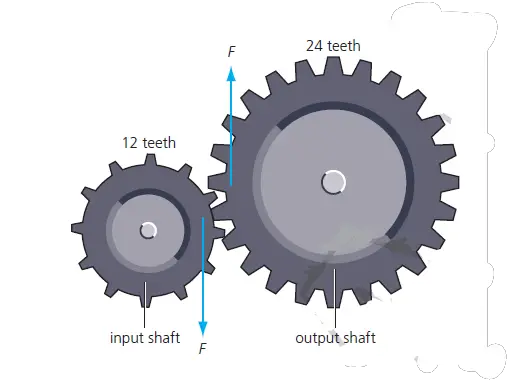# Gear as a Simple Machine

Last updated on June 14th, 2023 at 03:20 pm

A gear is a wheel and axle with the wheel having teeth around its rim. When the teeth of two gears interlock, the turning of one gear causes the other gear to turn.

Many machines make use of gears. Gears can be used to increase or decrease the rotational effects of a force.figure 1: Where gear teeth touch, equal and opposite forces act. Say In this case, the output gear wheel has twice the radius of the input wheel.

Say In this case, the output gear wheel has twice the radius of the input wheel.

The turning effect from the input shaft = F × r
The turning effect from the output shaft = F × 2r

The teeth of the input and output wheels exert the same force, F, on each other. However, because the output wheel has twice the radius of the input wheel, the turning effect from the output shaft is twice as big as the turning effect applied by the input shaft.

See this: gear numerical Solved

## features of a gear

• When two gears of different sizes are interlocked, they rotate at different rates.
• Each rotation of the larger gear causes the smaller gear to make more than one rotation.
• If the input force is applied to the larger gear, the output turning effect exerted by the smaller gear is less than the input turning effect exerted by the input gear.
• Gears also may change the direction of the force, as shown in Figure 1. When the larger gear is rotated clockwise, the smaller gear rotates counterclockwise.